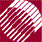ECE532 Biomedical Optics © 1998 Steven L. Jacques, Scott A. Prahl Oregon Graduate Institute

Save bin arrays in output files.

The final step after all photons have been launched is to save the results into output files for plotting by the user's favorite graphics program.

The output file is called "mc321.out" and is assigned to a pointer called "target". The output file is organized as a 3-line header with four columns:

number of photons = 10000.000000
bin size = 0.03000 [cm]
last row is overflow. Ignore.
r [cm]  	 Fsph [1/cm2] 	 Fcyl [1/cm2] 	 Fpla [1/cm2]
0.01500 	 3.431e+02  	 1.570e+01  	 3.983e+00
0.04500 	 3.773e+01  	 5.093e+00  	 2.667e+00

The bin arrays Csph[ir], Ccyl[ir] and Cpla[ir] hold the amount of absorbed photon weight. Dividing that weight by the total number of photons and by the shell volume yields the concentration of absorbed photons in a bin relative to the total number of photons launched [cm-3]. The shell volume is different for each of the dimensional geometries (spherical, cylindrical, planar).

Dividing each bin concentration by the absorption coefficient µa [cm-1] (mua) yields the relative fluence rate F/P [cm-2], where F [W cm-2] is the fluence rate and P [W] is the source power.

That's it !

/**** SAVE
Convert data to relative fluence rate [cm^-2] and save to file called "mcmin321.out".
*****/
target = fopen("mc321.out", "w");

fprintf(target, "number of photons = %f\n", Nphotons);
fprintf(target, "bin size = %5.5f [cm] \n", dr);
fprintf(target, "last row is overflow. Ignore.\n");

/* print column titles */
fprintf(target, "r [cm] \t Fsph [1/cm2] \t Fcyl [1/cm2] \t Fpla [1/cm2]\n");

/* print data:  radial position, fluence rates for 3D, 2D, 1D geometries */
for (ir=0; ir<=NR; ir++) {
/* r = sqrt(1.0/3 - (ir+1) + (ir+1)*(ir+1))*dr; */
r = (ir + 0.5)*dr;
shellvolume = 4.0*PI*r*r*dr; /* per spherical shell */
Fsph = Csph[ir]/Nphotons/shellvolume/mua;
shellvolume = 2.0*PI*r*dr;   /* per cm length of cylinder */
Fcyl = Ccyl[ir]/Nphotons/shellvolume/mua;
shellvolume = dr;            /* per cm2 area of plane */
Fpla =Cpla[ir]/Nphotons/shellvolume/mua;
fprintf(target, "%5.5f \t %4.3e \t %4.3e \t %4.3e \n", r, Fsph, Fcyl, Fpla);
}

fclose(target);

to next page | Chapter 4 | Course | Home

©1998, Steven L. Jacques, Scott A. Prahl, Oregon Graduate Institute, Oregon Medical Laser Center